Most Affordable JEE | NEET | 8,9,10 Preparation by Kota's Top IITian Doctor Faculties

# NCERT Class 11 Maths Chapter 8 Exercise 8.1 Solutions - Binomial Theorem - Free PDF Download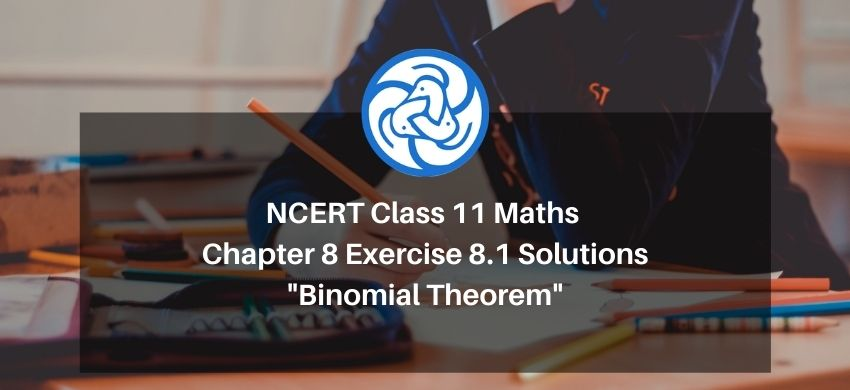Hey, are you a class 11 Student and Looking for Ways to Download Class 11 Maths Chapter 8 Exercise 8.1 Solutions? If Yes then you are at the right place.

All the solutions of Class 11 Maths Chapter 8 exercise 8.1 is prepared by Kota’s top IITian’s Faculties by keeping Simplicity in mind.

If you want to score high in your class 11 Maths Exam then it is very important for you to have a good knowledge of all the important topics, so to learn and practice those topics you can use eSaral NCERT Solutions.

So, without wasting more time Let’s start.

### Download The PDF of NCERT Class 11 Math Chapter 8 Exercise 8.1 Solutions "Binomial Theorem"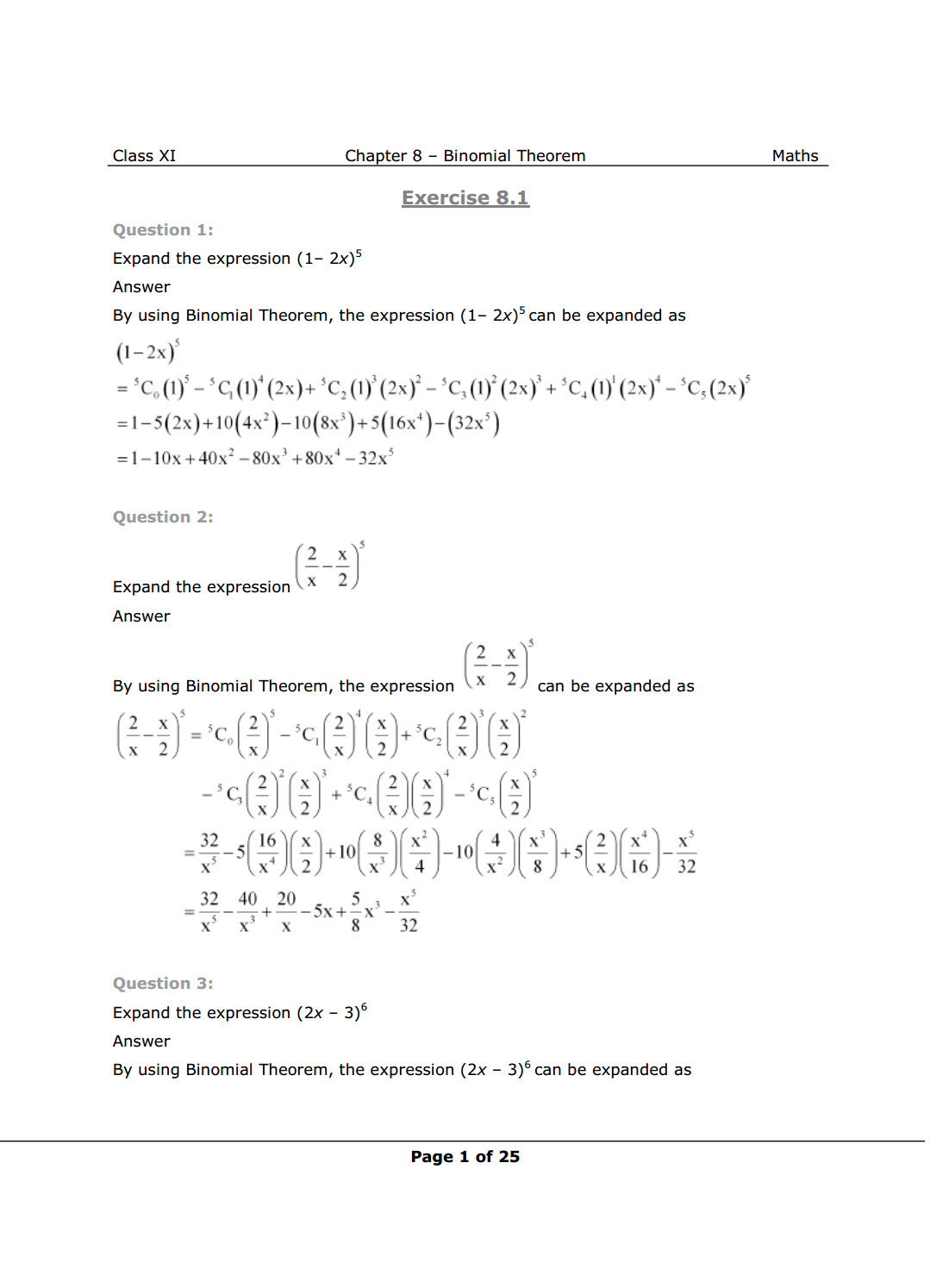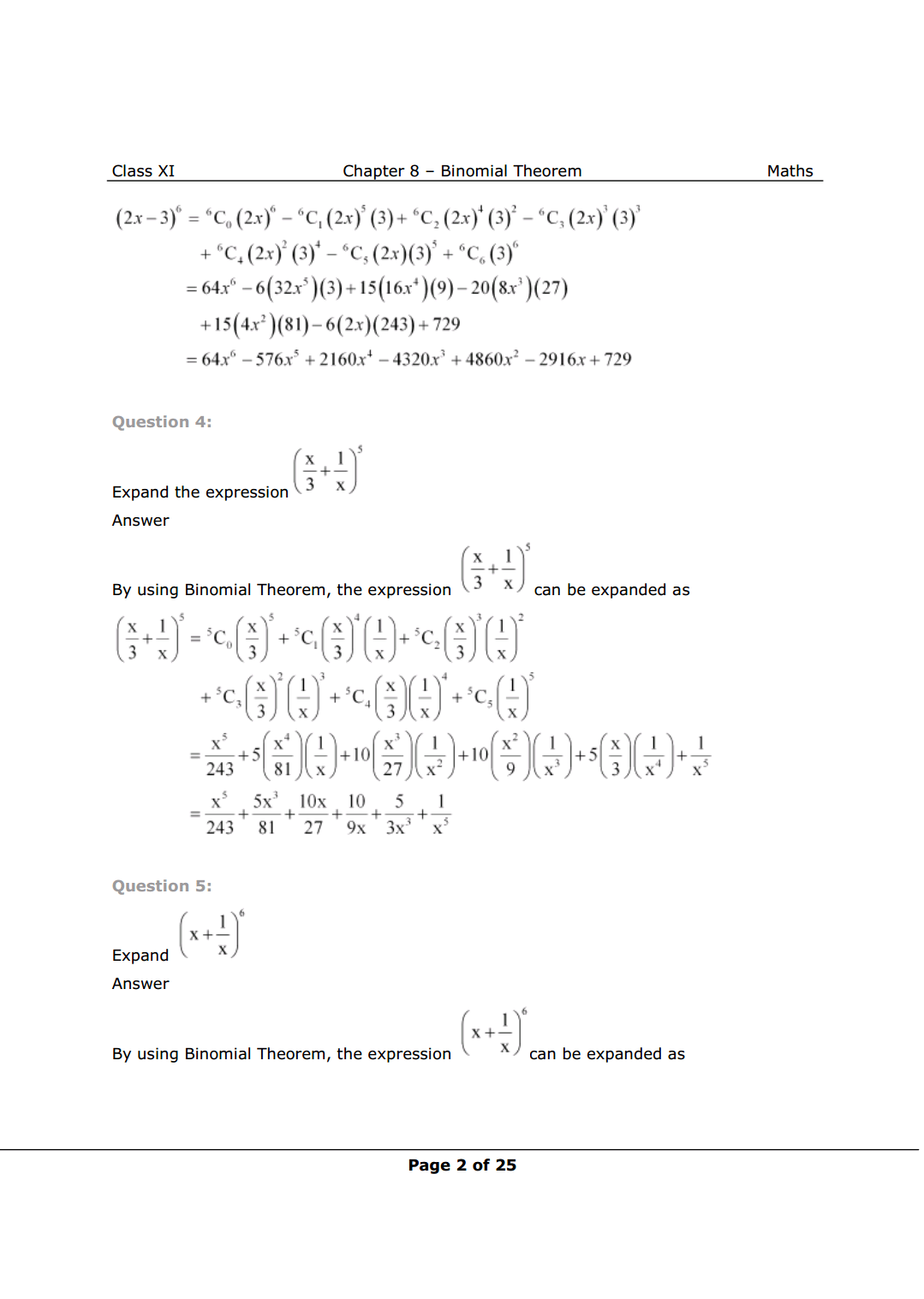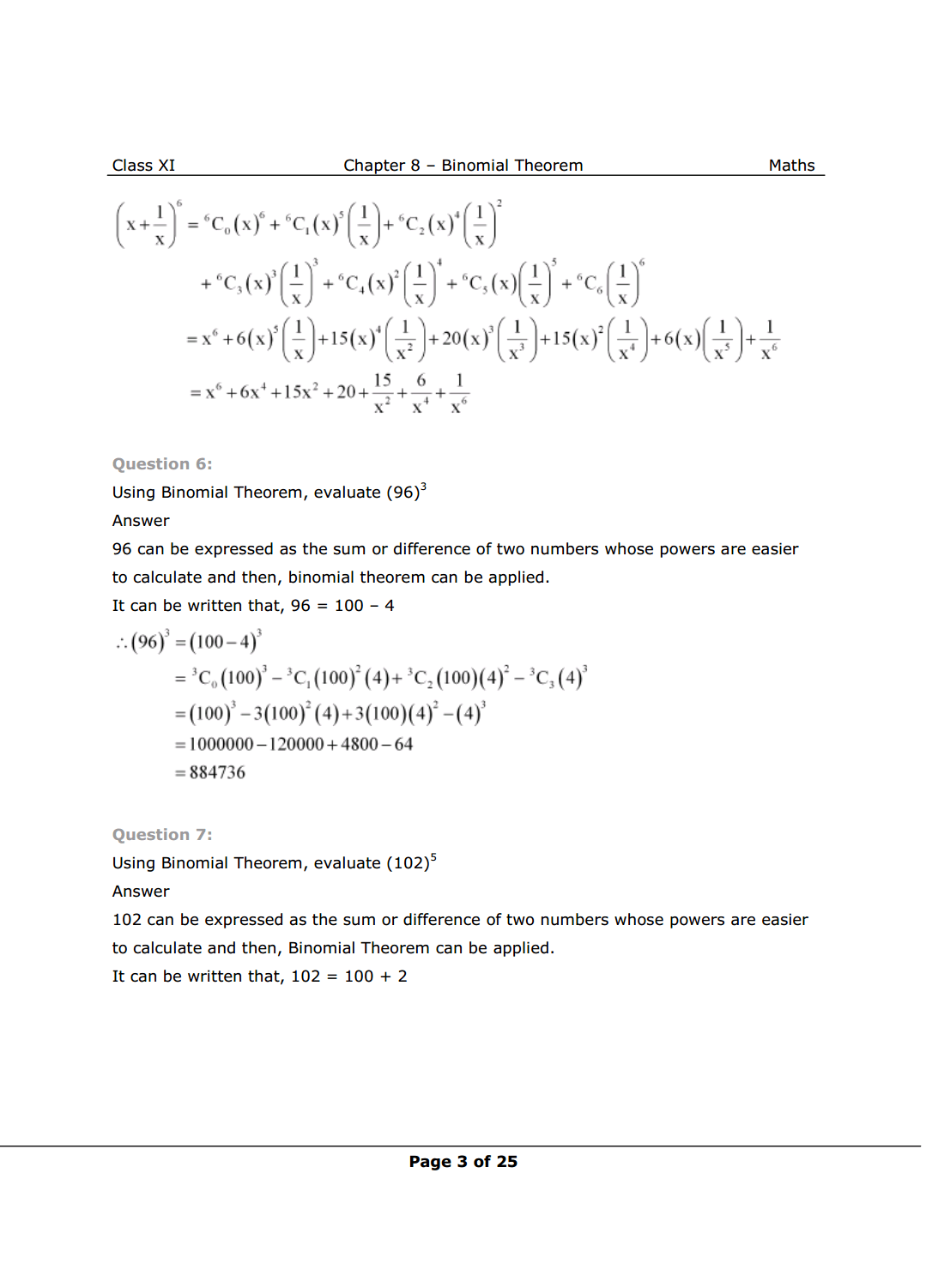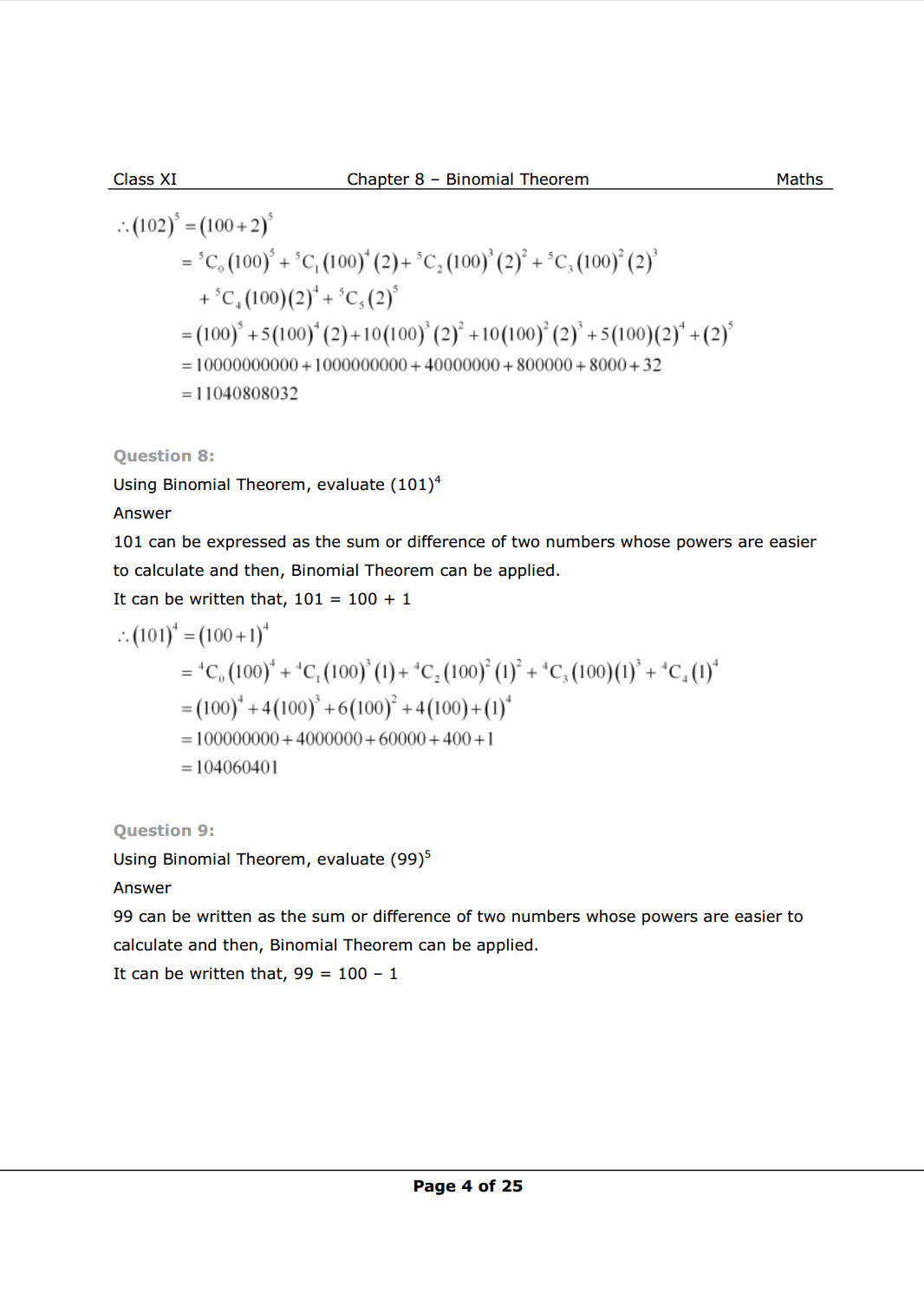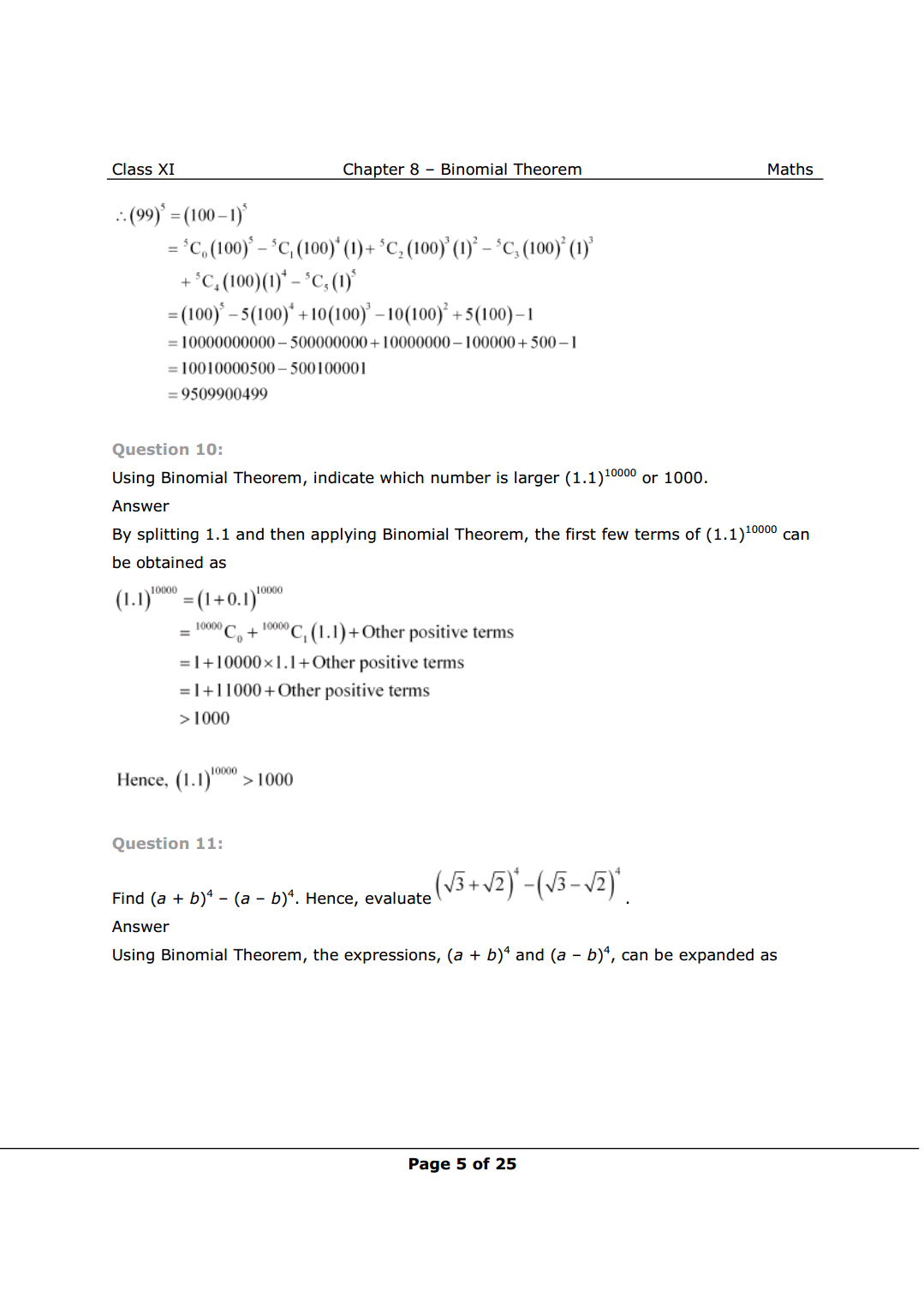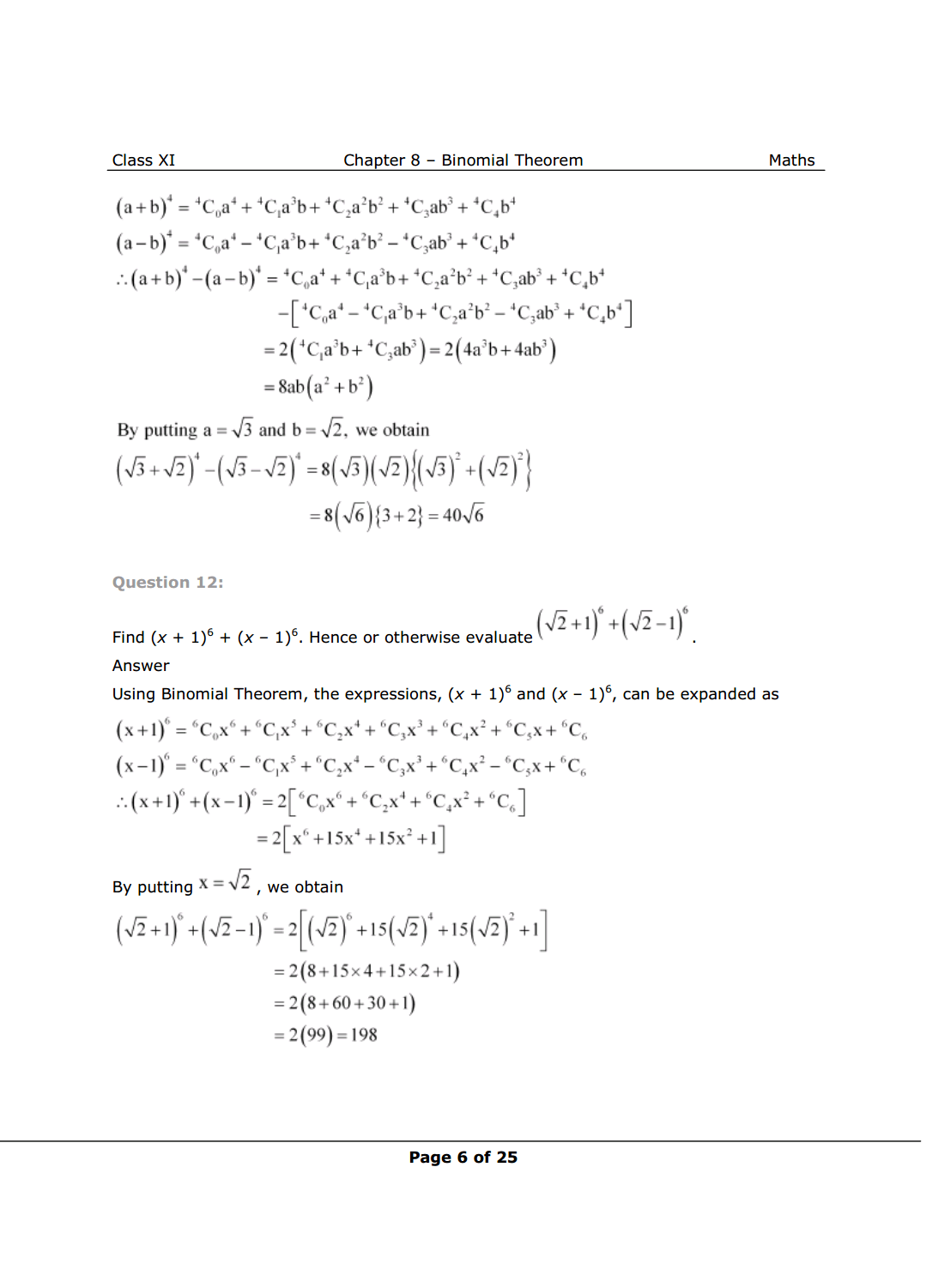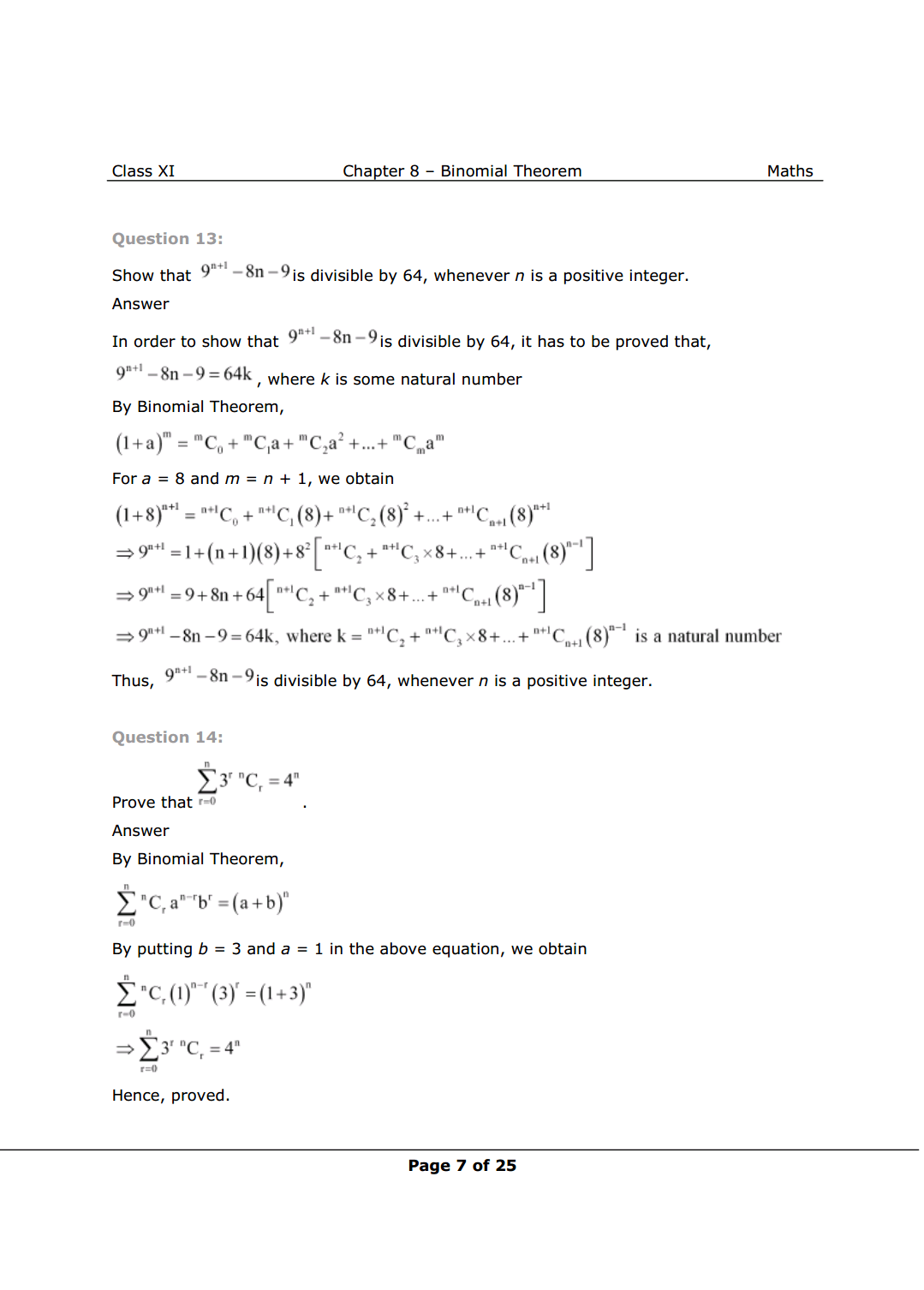NCERT Class 11 Math Book Free PDF

NCERT Class 11 Maths Exemplar Free PDF

Complete Solutions for Class 11 Maths chapter 8  Free PDF

Class 11 maths chapter 7 exercise 7.1 Solutions Free PDF

Class 11 maths chapter 7 exercise 7.2 Solutions Free PDF

Class 11 maths chapter 7 exercise 7.3 Solutions Free PDF

Class 11 maths chapter 7 exercise 7.4 Solutions Free PDF

Class 11 maths chapter 7 Miscellaneous exercise Solutions Free PDF

If you have any Confusion related to Class 11 Math Chapter 8 Exercise 8.1 Solutions then feel free to ask in the comments section down below.

To watch Free Learning Videos on Class 11 by Kota’s top Faculties Install the eSaral App